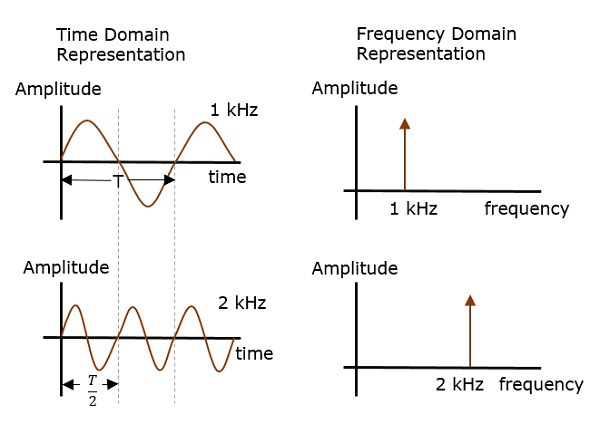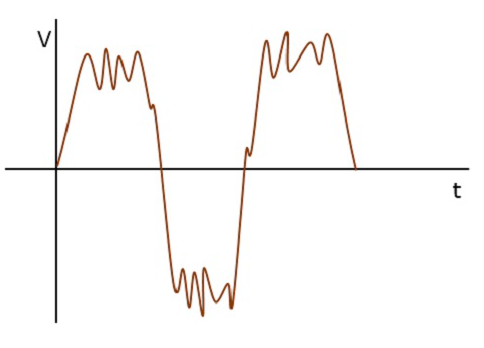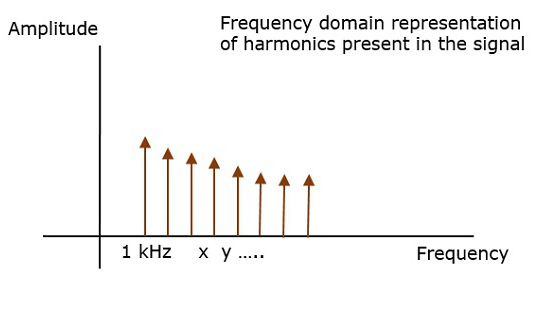# Analyzing Signals

To analyze a signal, it has to be represented. This representation in communication systems is of two types −

• Frequency domain representation, and
• Time domain representation.

Consider two signals with 1 kHz and 2 kHz frequencies. Both of them are represented in time and frequency domain as shown in the following figure.Time domain analysis, gives the signal behavior over a certain time period. In the frequency domain, the signal is analyzed as a mathematical function with respect to the frequency.

Frequency domain representation is needed where the signal processing such as filtering, amplifying and mixing are done.

For instance, if a signal such as the following is considered, it is understood that noise is present in it.The frequency of the original signal may be 1 kHz, but the noise of certain frequency, which corrupts this signal is unknown. However, when the same signal is represented in the frequency domain, using a spectrum analyzer, it is plotted as shown in the following figure.Here, we can observe few harmonics, which represent the noise introduced into the original signal. Hence, the signal representation helps in analyzing the signals.

Frequency domain analysis helps in creating the desired wave patterns. For example, the binary bit patterns in a computer, the Lissajous patterns in a CRO, etc. Time domain analysis helps to understand such bit patterns.

## Useful Video Courses

Video

#### Basic Principles of Organic Chemistry

75 Lectures 5 hours

Video

#### Ionic & Angular JS: Principles Of Mobile and Web Development

16 Lectures 2.5 hours

Video

#### Social Media Marketing: Quick Guide of Basic Principles

9 Lectures 1 hours

Video

#### Principles Of Success – Growth Mindset

12 Lectures 1 hours

Video

#### Principles of Financial Accounting

12 Lectures 1.5 hours

Video

#### Applied Finance: Finance Principles

45 Lectures 2 hours